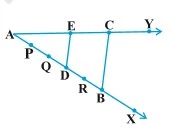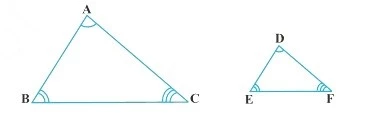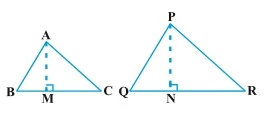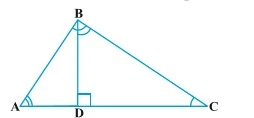# Triangles NCERT Solutions : Class 10 Maths

## NCERT Solutions for Class 10 Maths : TrianglesFilter
Filters :
Classes
•
•
•
•
•
•
•
Chapters
•
•
•
•
•
•
•
•
•
•
• 5 More
Exercises
•
•
•
•
•
•
•

### NCERT Class 10 | TRIANGLES | Exercise 06 | Question No. 05

In figure, Ad is a median of a triangle ABC and A M_|_B C . Prove that: (i) A C^2=A D^2+B C""""dot""""D M""+ ((B C)/2)^2 (ii) A B^2=A D^2-B C""""dot""""D M""+ ((B C)/2)^2 (iii) A C^2+A B^2=2A D^2+ 1/2B C^2Doubtnut one of the best online education platform provides free NCERT Solutions of Maths for Class 10 Triangles which are solved by our maths experts as per the NCERT (CBSE) guidelines. We provide the solutions as video solutions in which concepts are also explained along with answer which will help students while learning , preparing for exams and in doing homework. These Solutions will help to revise complete syllabus and score more marks in examinations.

Get here free, accurate and comprehensive NCERT Solutions for Class 10 Maths Triangles which have been reviewed by our maths counsellors as per the latest edition following up the CBSE guidelines. We provide video solutions in which solutions to all the questions of NCERT Class 10 Maths textbook are explained in step by step and detailed way.

## The Topics of the Chapter Triangles are :

INTERNAL AND EXTERNAL ANGLE BISECTORS OF A TRIANGLE

PYTHAGORAS THEOREM

CRITERIA FOR SIMILARITY OF TRIANGLES

PROPERTIES OF SIMILAR TRIANGLE

SIMILAR TRIANGLES AND THEIR PROPERTIES

MORE ON BASIC PROPORTIONALITY THEOREM

MORE ON CHARACTERISTICS PROPERTIES

AREAS OF TWO SIMILAR TRIANGLES

INTERNAL AND EXTERNAL BISECTORS OF AN ANGLE OF A TRIANGLE

SIMILARITY

It contains these exercises along with solved examples. All the exercises are solved in the video. Select the exercise to view the solutions exercisewise of the Chapter Triangles:

## NCERT Solutions Class 10 Maths Chapter Triangles Exercises:

We have covered all the exercises and also Solved examples in the videos. Along with the practise exercise Students should also practise solved examples to clear the concepts of the Triangles. If incase you have any doubt you can watch the solutions for the given questions.Watch the solutions for the given questions in which it is also explained in the video steps to solve the questions along with answers.

This chapter deals with the geometrical figure triangle and its properties. Here, all important topics like similar figures, similarity of Triangles, criteria for similarity of Triangles and areas of Triangles are discussed. This helps students to get the perfect idea on how to draw the Triangles accurately so as to find the right result out of it. Doubtnut has made it possible for the students to gather all important details that lead them to clearing their queries.

During exams, students find it quite difficult to attempt certain questions in Maths. This is because of the inadequate knowledge that the students get from their NCERT textbooks due to lack of ample solved questions. It has been seen that these books fail to provide in-depth understanding of the different topics. So, this leads to a lot of problem in getting the right idea on how to work on the different solutions which, in turn, leads to low marks in Maths. So, with the hope of achieving success in the exams, they resort to different reference books or guides. This helps them to get some good explanation on the different topics.

The expert team of tutors of Doubtnut has made learning much easy for class 10 CBSE students. This has been possible from the best approach that has been taken to clear all the queries by the experts. With the best Class 10 Maths NCERT Solutions provided by them, it becomes quite possible to get all the perfect idea on how to attempt the different questions in the best way. Students can look forward to the step by step procedure which has been provided by them so that the students do not find any sort of problem in dealing with different questions. So, students who wish to get higher marks in Maths should definitely look forward to the most useful study materials provided by Doubtnut.

## Topics and Subtopics included in NCERT Solutions for Class 10 Maths Chapter 6 Triangles

### Introduction

In your earlier classes, you have learned about triangles and many of its properties. Let’s recall that two figures are said to be congruent if they have the same size and shape. In this chapter we will discuss about the figures which have the same shape but not of the same size.

### Similar Figures

All circles with the same radii are known to be congruent where all equilateral triangles with the same side lengths are congruent. It is to be noted that all congruent figures are similar but the similar figures need to be congruent. If there are two photographs of the same size and that too of the same person one at the age of 20 years and the other at the age of 40years, what would you say about it? Do you think that these photographs are similar? When it comes to the size of the photographs, they are of the same size but they are not of the same shape.

### Similarity of Triangles

It is to be noted that triangle is also a polygon. So, when it comes to the similarity of two triangles, we can state some conditions like:

(i) when their corresponding angles are found to be equal and

(ii) when their corresponding sides are found to be in the same ratio (or proportion).

Equiangular triangles are said when corresponding angles of two triangles are equal. The ratio of any two corresponding sides in two equiangular triangles is always the same.

Let’s discuss the Basic Proportionality Theorem with the help of the following activity:

Draw any angle XAY and on its one arm AX, mark points (say five points) P, Q, D, R and B such that AP = PQ = QD = DR = RB.Now, through B, draw any line intersecting arm AY at C

Also, through the point D, draw a line parallel to BC to intersect AC at E. Do you observe from your constructions that AD =3

DB    2

Measure AE and EC. What about AE? Observe that AE is equal to 3

EC                            EC                    2

Thus, you can say that in âˆ† ABC, DE || BC and AD = AE

DB    EC

This is because of the Basic Proportionality Theorem.

### Criteria for Similarity of Triangles

We have seen that two triangles are similar when their corresponding angles are equal and their corresponding sides are in the same ratio.

That is, in âˆ† ABC and âˆ† DEF, if

(i )  ∠ A = ∠ D, ∠ B = ∠ E, ∠ C = ∠ F and

(ii) AB = BC = CA then two triangles are similar

DE     EF     FDHere, you can see that A corresponds to D, B corresponds to E and C corresponds to F. Symbolically, we write the similarity of these two triangles as ‘âˆ† ABC ~ âˆ† DEF’ and read it as ‘triangle ABC is similar to triangle DEF’. The symbol ‘~’ stands for ‘is similar to’. Recall that you have used the symbol ‘≅’ for ‘is congruent to’ in Class IX.

Now a natural question arises: For checking the similarity of two triangles, say ABC and DEF, should we always look for all the equality relations of their corresponding angles (∠ A = ∠ D, ∠ B = ∠ E, ∠ C = ∠ F) and all the equality relations of the ratios of their corresponding sides AB = BC = CA ? You may recall that

DE     EF     FD

in Class IX, you have obtained some criteria for congruency of two triangles involving only three pairs of corresponding parts (or elements) of the two triangles. Here also, we can make an attempt to arrive at certain criteria for similarity of two triangles involving relationship between less number of pairs of corresponding parts of the two triangles, instead of all the six pairs of corresponding parts.

### Areas of Similar Triangles

It has been learned that in two similar triangles, the ratio of their corresponding sides is the same. Do you have any idea as to whether there is any relationship between the ratio of their areas and the ratio of the corresponding sides? It is known that area is measured in square units. So, it can be expected that this ration is the square of the ratio of their corresponding sides. Let’s have a look at it.

Theorem: The ratio of the areas of two similar triangles is equal to the square of the ratio of their corresponding sides.Proof:  We are given two triangles ABC and PQR such that âˆ† ABC ~ âˆ† PQR

We need to prove that ar (ABC) = (AB)2 = (BC)2 = (CA)2

ar (PQR)    (PQ)2    (QR) 2    (RP) 2

In order to find the areas of the two triangles, we need to draw altitudes AM and PN of the triangles.

Now, ar (ABC)= 1BC x AM

2

ar (PQR)= 1QR x PN

2

So,    ar (ABC) = 1/2x BC x AM = BC x AM     (1)

ar (PQR)    1/2 x QR x PN    QR x PN

Now, in âˆ† ABM and âˆ† PQN,

∠ B = ∠ Q (As âˆ† ABC ~ âˆ† PQR)

and   ∠ M = ∠ N (Each is of 90°)

So,   âˆ† ABM ~ âˆ† PQN (AA similarity criterion)

Therefore,          AM = AB                                   (2)

PN     PQ

Also, âˆ† ABC ~ âˆ† PQR (Given)

So,                   AB = BC = CA                            (3)

PQ     QR   RP

Therefore,    ar (ABC) = AB x AM     [From (1) and (3)]

ar (PQR)    PQ   PN

= AB x AM     [From

PQ   PQ

= (AB)2

(PQ)2

Now using (3), we get ar (ABC) = (AB)2 = (BC)2 = (CA)2

ar (PQR)      (PQ) 2    (QR)2     (RP)

### Pythagoras Theorem

From your earlier classes, you have learned Pythagoras Theorem. By using the concept of similarity of triangles, we shall prove this theorem.Let us take a right triangle ABC, right angled at B. Let BD be the perpendicular to the hypotenuse AC

You may note that in âˆ† ADB and âˆ† ABC

∠ A = ∠ A and ∠ ADB = ∠ ABC

So,                                            âˆ† ADB ~ âˆ† ABC       (1)

Similarly, âˆ† BDC ~ âˆ† ABC       (2)

So from (1) and (2), triangles on both sides of the perpendicular BD are similar to the whole triangle ABC.

Also, since                                âˆ† ADB ~ âˆ† ABC

And                                          âˆ† BDC ~ âˆ† ABC

So,                                           âˆ† ADB ~ âˆ† BDC

Thus this theorem can be also applied in proving the Pythagoras Theorem.

## Significance of Doubtnut for Class 10 students

Class 10 students can truly benefit a lot from Doubtnut’s video tutorials. This is because of the simplicity of languages that have been used by the experts in preparing the different topics. By getting hold of the right NCERT Class 10 Maths Solutions Chapter 6, things become much easier for them to get their concepts cleared. Well, let us have a look at the significance of Doubtnut’s online study materials that would make you get the perfect idea about it.

• Downloadable solutions:  Students can download the NCERT maths solutions in pdf format. They can then try to access it from anywhere. So, this proves to be quite flexible where they do not have to fix any particular time for it. Therefore, with the right decision made by the students to get the accurate solutions from Doubtnut, it helps them to stay quite confident in exams. This is because they can find it quite easy to learn any topics in the best manner.
• No need to pay any fees: The online study material that the students get from Doubtnut are free of cost.  So, there is no need to pay any fees for it that proves to be an added advantage to them.  Students also do not have to search elsewhere for their solutions as they can get it online from the right experts.
• Learn from experienced tutors: Learning the right technique from the best in class experts can really help a lot to solve even the most difficult questions. So, it becomes possible to achieve success in the exams without any worry at all.

Share this on :-